# vs.eyeandcontacts.com

## Chapter 11 - Force and Pressure Notes

1. Force
Force is push or pull of an object. Example: An object has to be moved, it has to be pushed or pulled.

2. Forces are due to interactions
An interaction of one object on another object results in a force between the two objects. Example: If a man stands behind a car, the car doesn’t move. If he pushes the car, he applies force and the car begins to move in the direction of applied force.

3. Exploring forces
→ If two forces act on a body in the same direction, the net force is the sum of 2 forces.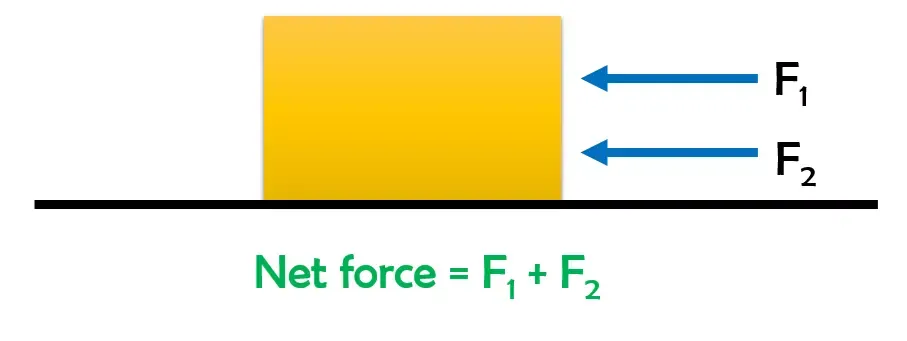→ If two forces act on a body in opposite directions, the net force is the difference between the two forces.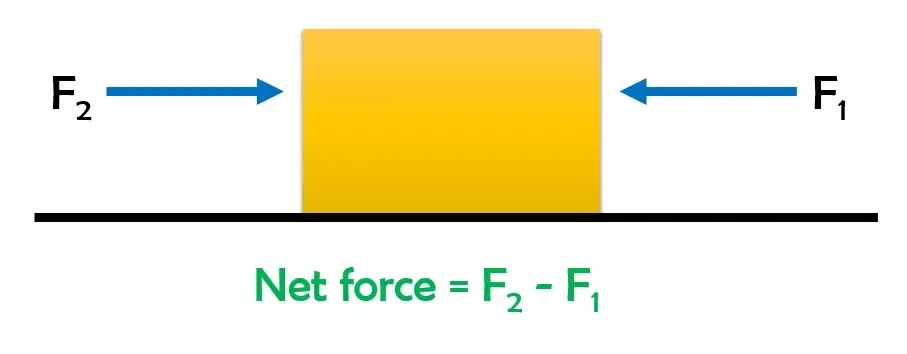The effect on the object depends on the net force acting on it.

4. Effects of force on an object:
→ Force can make an object to move from rest.
→ Force can stop a moving object.
→ Force can change the speed of an object if it is moving.
→ Force can change the direction of a motion of a moving object.
→ Force can change the shape of an object. Force may cause one or more of these effects.

5. Types of forces
There are two main types of forces. They are
Contact forces
Non-contact forces
• Contact forces are forces exerted by one object on another when they are in contact with each other. Contact forces are of two types. They are:
→ Muscular force
→ Force of friction (Friction or Frictional Force)

• Non-contact forces are forces exerted by one object another without any contact with each other. Non-contact forces are of three types. They are:
→ Magnetic force
→ Electrostatic force
→ Gravitational force (Gravity or Force of Gravity)

6. Muscular force
Muscular force is the force exerted by the muscles of our body. We use muscular force for our various activities. Animals like bullocks, horses, camels, donkeys, elephants also use muscular force to perform various tasks.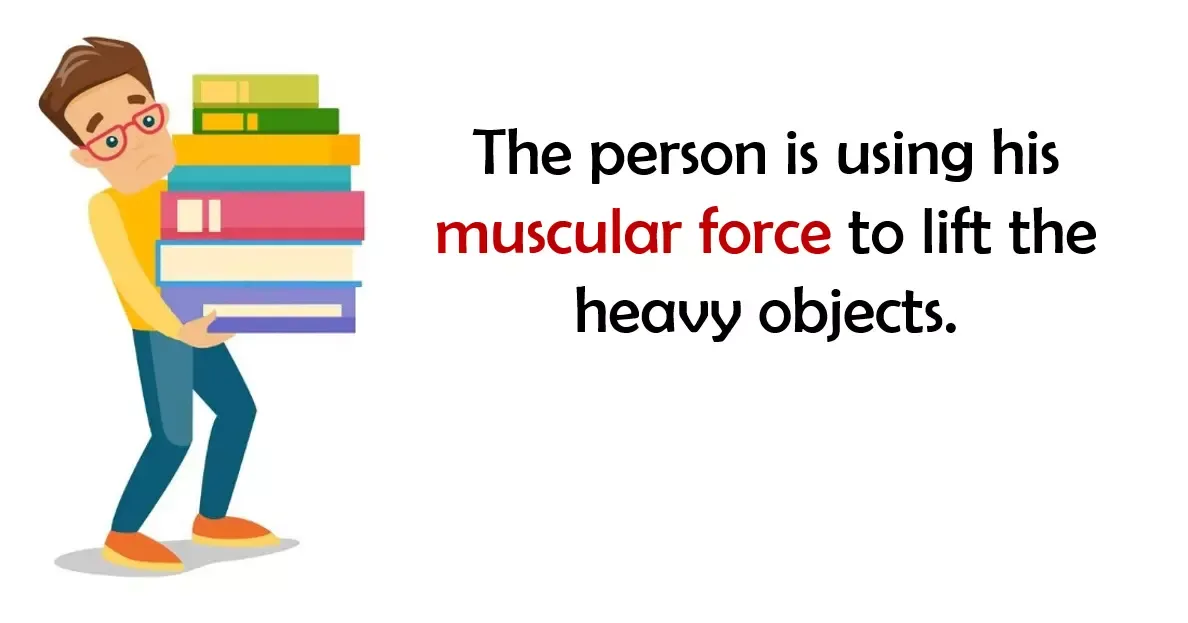7. Friction
It is also known as Frictional Force and Force of Friction. Force of friction is the force which opposes the motion of an object over a surface.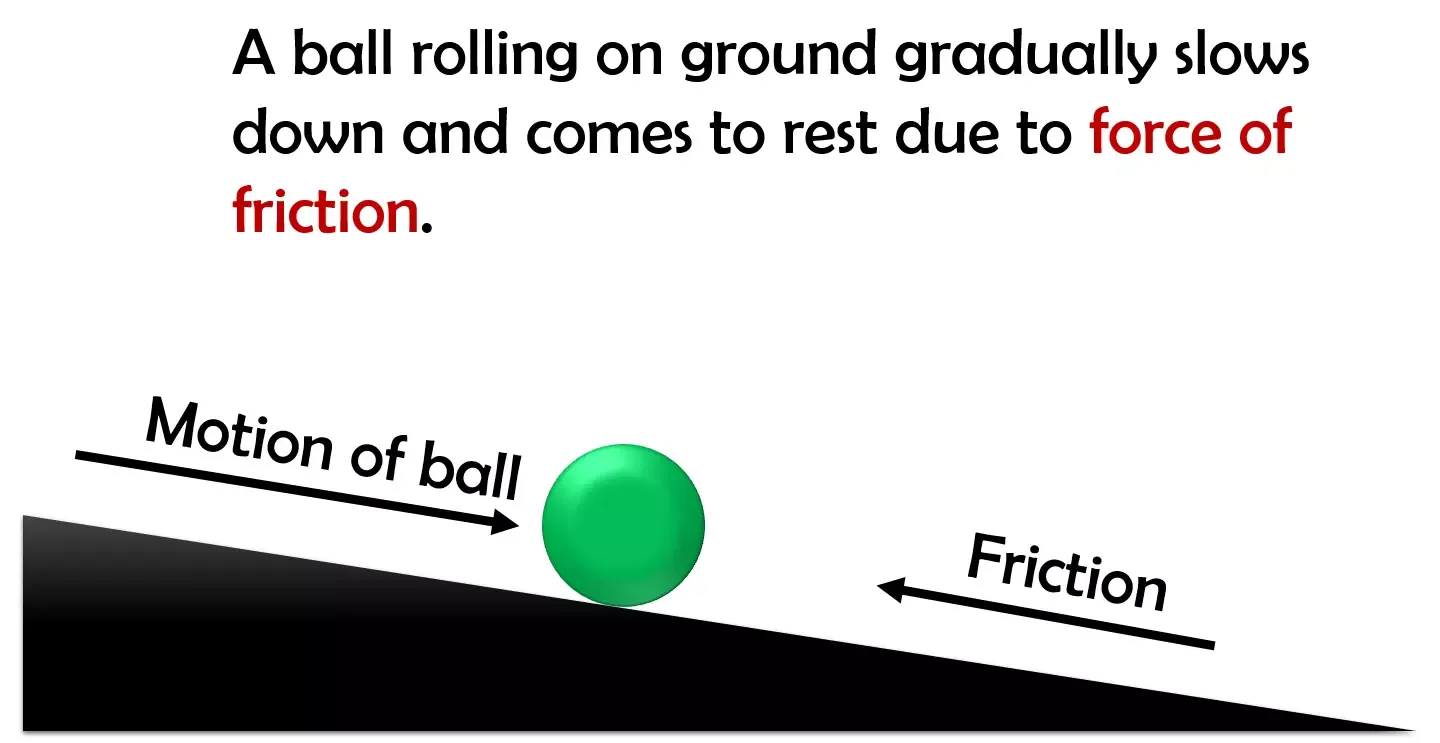Example: A ball rolling on ground gradually slows down and comes to rest due to force of friction. If we stop pedalling a bicycle, it gradually slows down and comes to a stop due to force of friction.

8. Magnetic force
Magnetic force is the force exerted by magnets.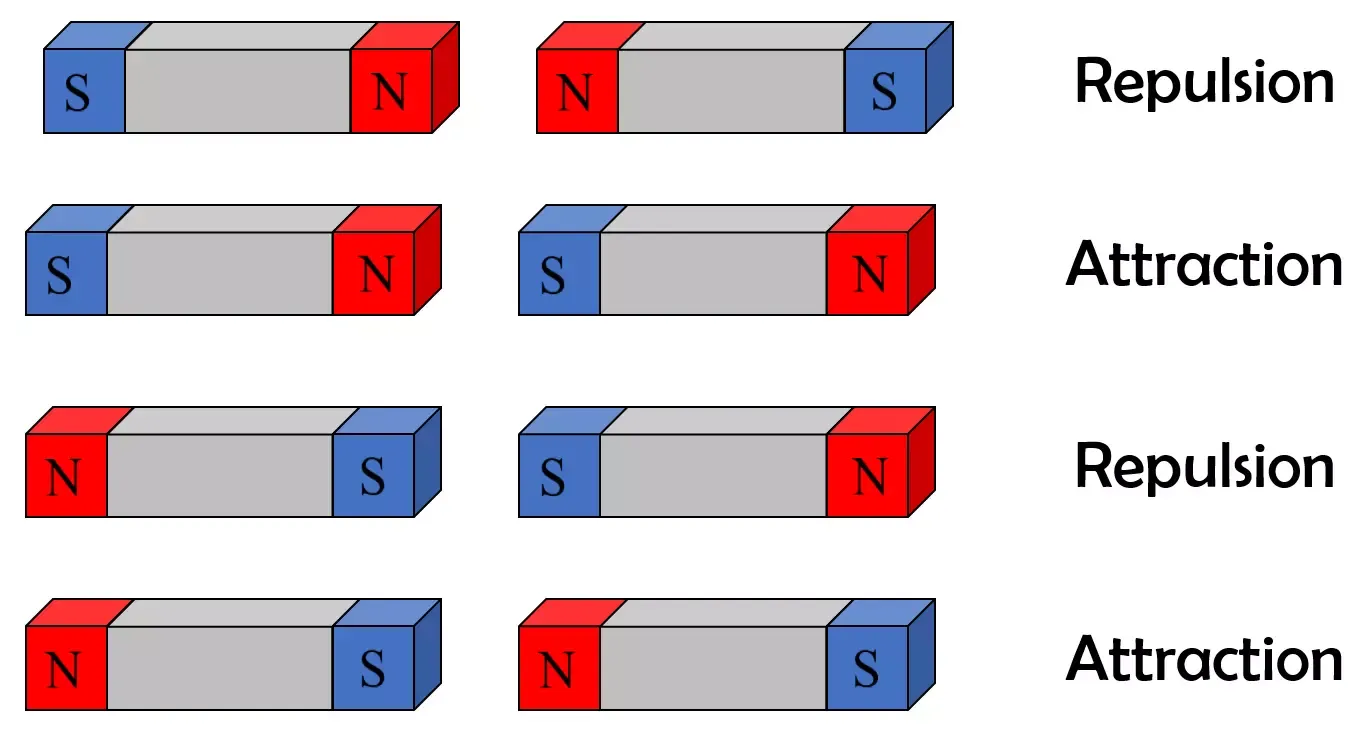Example: Force of attraction and repulsion between two magnets. Force exerted by a magnet on a piece of iron or steel.
North and North poles repel each other (Repulsion).
North and South poles attract each other (Attraction).
South and South poles repel each other (Repulsion).
South and North poles attract each other (Attraction).

9. Electrostatic force
Electrostatic force is the force exerted by a charged body on another charged or uncharged body.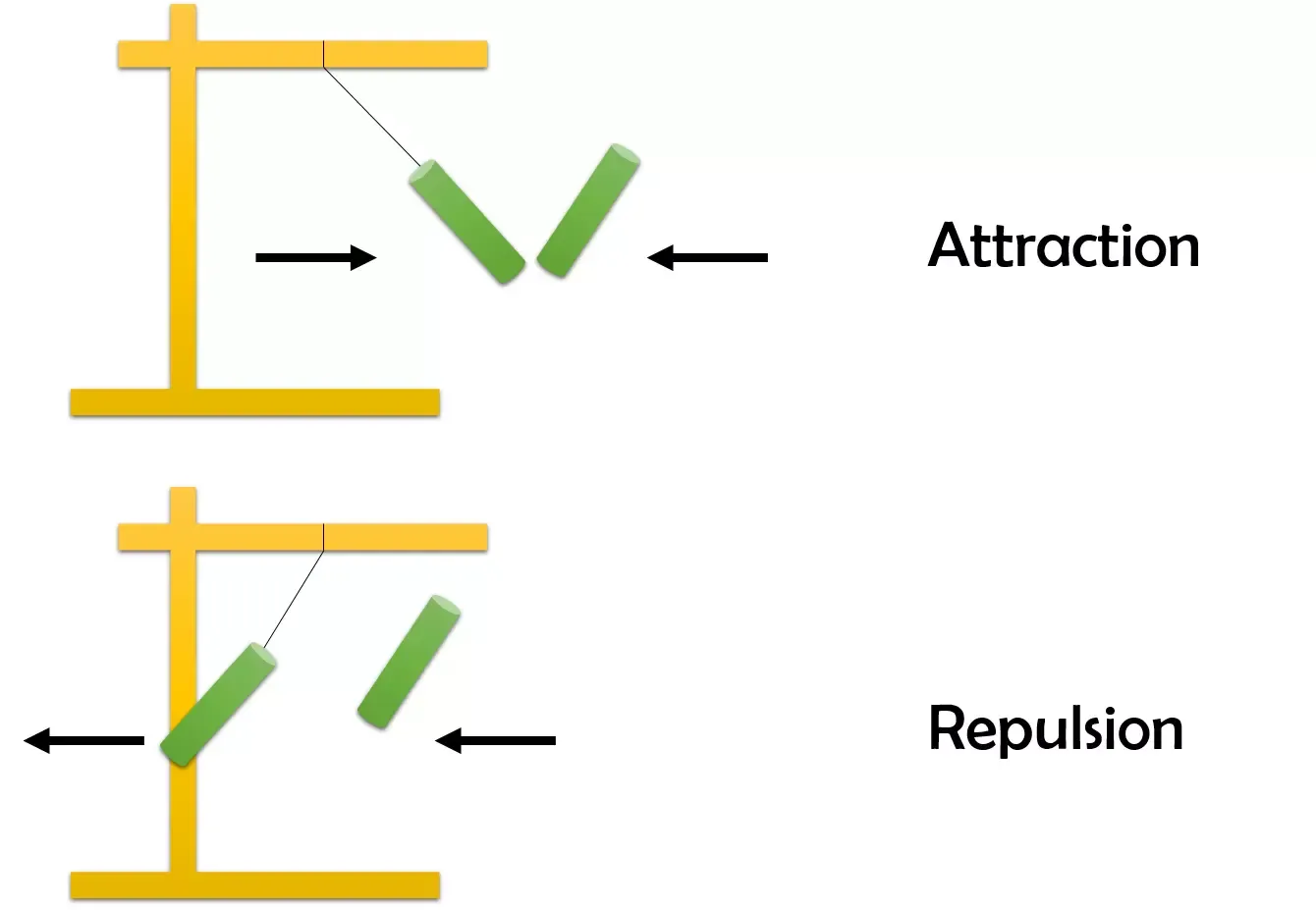Example: A plastic straw charged by rubbing with paper attracts a suspended plastic straw. A plastic straw charged by rubbing with paper repels a suspended plastic charged by rubbing with paper.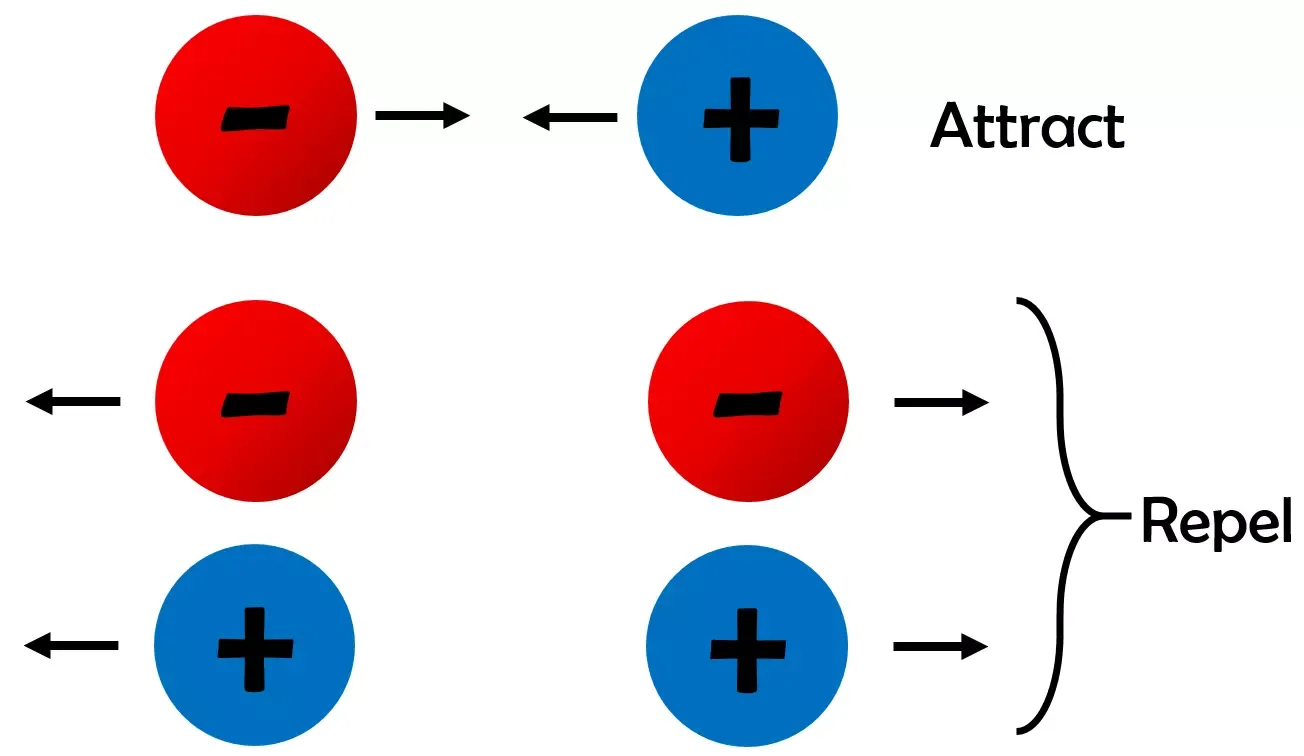10. Gravitational Force
It is also known as Gravity and Force of Gravity. Gravitational force is the force exerted by the earth on all objects. It pulls all objects towards the Earth.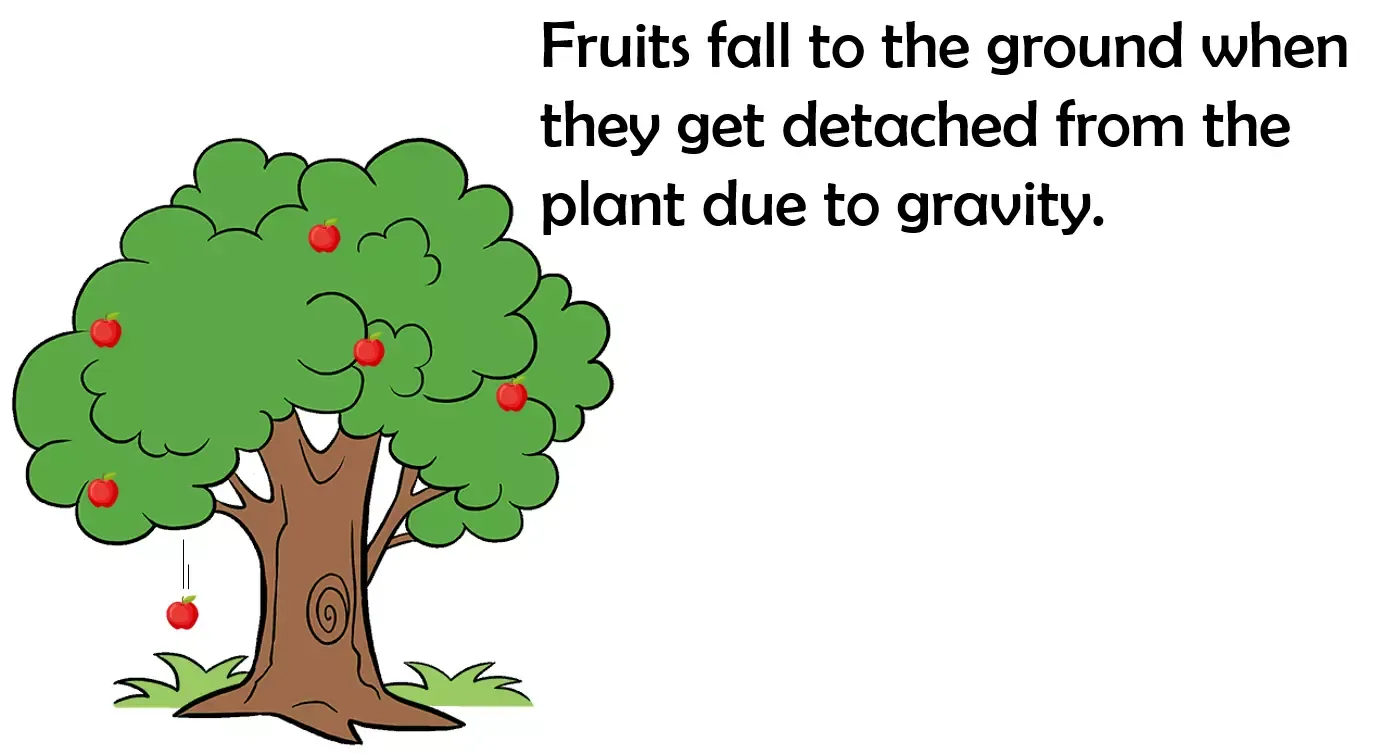Example: A coin or pen falls down when it slips off your hand. A ball thrown upward falls down. Leaves and fruits fall to the ground when they get detached from the plant. Water in rivers flow downwards.

11. Pressure
Pressure is the force acting on unit area of a surface.Pressure = Force/Area = N/m²
Unit = Nm²
If the area is smaller the pressure is more on the surface for the same force.
Example: It is easier to push a nail into a wooden plank by its pointed end than its head because the pressure is more. It is easier to cut vegetables with a sharp knife than a blunt knife because the pressure
is more. Shoulder bags have broad and not thin straps to reduce pressure.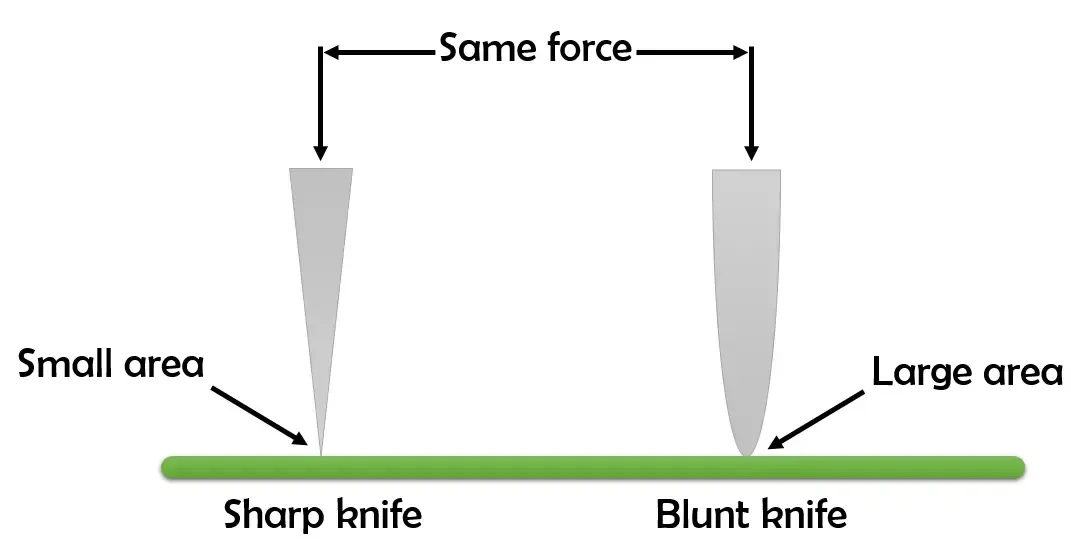12. The strength of a force is usually expressed by its magnitude.

13. State of Motion
The state of motion of an object is described by its speed and the direction of motion. The state of rest is considered to be the state of zero speed. An object may be at rest or in motion; both are its states of motion.

14. Porters place a round piece of cloth on their heads, when they have to carry heavy loads. By doing this, they increase the area of contact of the load with their head. So the pressure on their head is reduced and they find it easier to carry load.

15. Pressure exerted by liquids & gases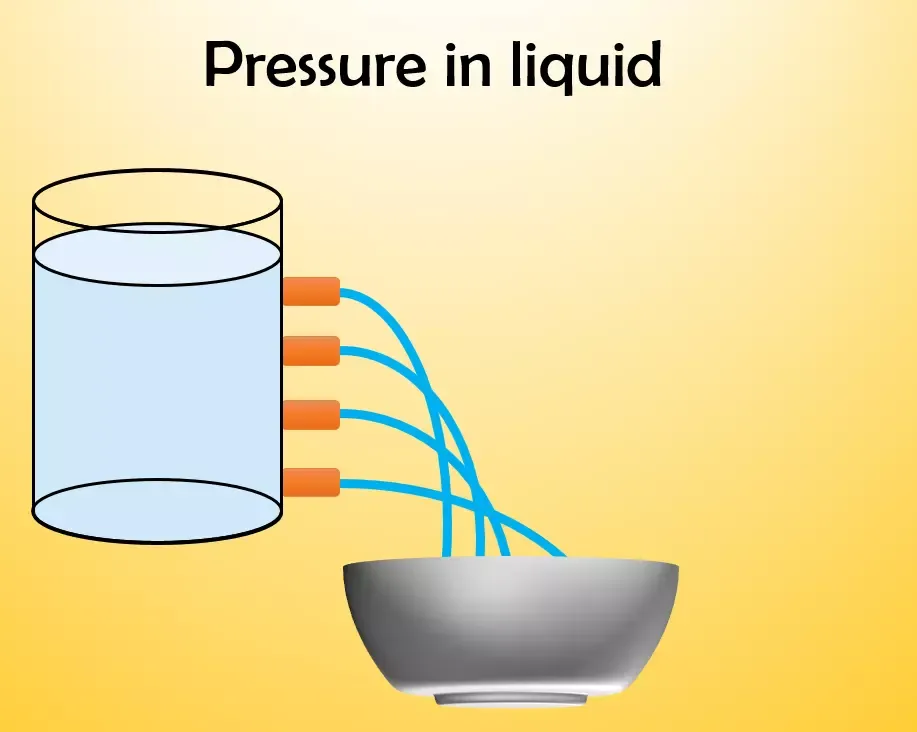→ Liquids exert pressure on the walls of a container.
→ Liquids exert pressure on the bottom of a container. It depends upon the height of the liquid column.
→ Liquids exert sideways pressure.
→ Liquids exert equal pressure at the same height.
→ Gases exert pressure on the walls of a container.

16. Atmospheric pressure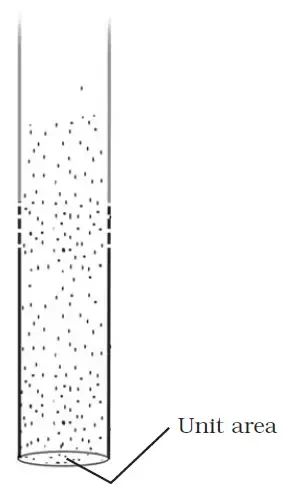Atmospheric pressure is the pressure of air around us. Atmospheric pressure is due to the weight of air. The pressure in our body balances the atmospheric pressure and that is why we don’t feel it.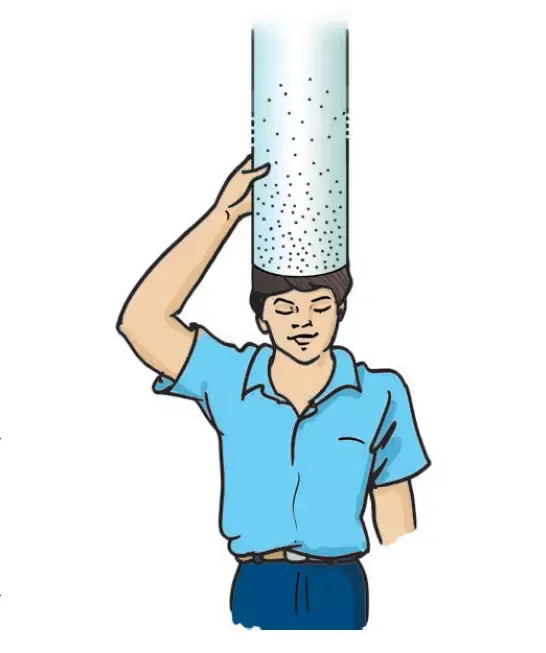17. The force due to air in a column of the height of the atmosphere and area 15 cm × 15 cm is nearly equal to the force of gravity on an object of mass 225 kg (2250N).

18. Otto von Guericke, a German scientist of the 17th century, invented a pump to extract air out of a vessel. With the help of this pump, he demonstrated dramatically the force of the air pressure. He joined two hollow metallic hemispheres of 51 cm diameter each and pumped air out of them. Then he employed eight horses on each hemisphere to pull them apart. So great is the force of air pressure that the hemispheres could not be pulled apart.

19. Push is the force exerted away from the body.

20. Pull is the force exerted towards the body.

21. Fluid pressure
Substances which can flow and has no fixes shape are called fluids.
Fluid pressure formula = pgh
p = fluid density
g = acceleration due to friction
h = fluid depth

→ Pressure inside the fluid increases with increase in depth and density of the fluid.

23. Thrust is the force that acts on a surface in a direction perpendicular to it.

24. Scalar quantity and Vector quantity
Scalar quantity is defined as the physical quantity with only magnitude and no direction. Example: Mass, speed, distance, time, area, volume, density, temperature.
Vector quantity is defined as the physical quantity that has both directions as well as magnitude. Example: Acceleration, Displacement, Momentum, Force.

25. When the airplane goes up, why do we feel our ears are blocked?
When an airplane climbs or descends, the air pressure changes rapidly. The eustachian tube often can't react fast enough, which causes the ears to block. Swallowing or yawning opens the eustachian tube and allows the middle ear to get more air, equalizing the air pressure.

26. SI unit of Pressure is Pascal.

27. The force that acts on an object perpendicular to its surface is called thrust.

28.
F = m × a
F =  force
m =  mass of an object
a =  acceleration

29. Why do mountaineers suffer from nose-bleeding at high altitudes?
Air pressure goes on decreasing as we go higher up, so at higher altitudes, the atmospheric pressure becomes less than the pressure exerted by the blood inside the body. This causes blood vessels burst and the nose starts bleeding.